Question

# 8. How many gram Na in 5.00g Na2SO4? A. 0.035 g                   B. 1.31 g

8. How many gram Na in 5.00g Na2SO4?

A. 0.035 g                   B. 1.31 g                     C. 1.62 g                     D. 0.97 g                     E. 0.81 g

9. A compound containing only carbon and hydrogen has   85.7 wt% C and    14.3 wt%H. Its molar mass is 56 amu. What is its molecular formula?

A. C4H8                       B. C2H4                       C. C2H                               D. CH2                                    E. CH

10. Balance the following reactions. No fractional coefficients, coefficients must be the lowest whole numbers.

a)         ____K   +   ____H2O     →   ____KOH +   ____H2

b)         ____H2O2      →   ____H2O + ____O2

c)         ____C2H6   +   ___ O2   →   ____CO2   + ____H2O

d)         ____KOH   + ____H3PO4 →    ____K3PO4   +   ____H2O

e)         ____C2H5OH + ____O2 → ____CO2 + ____H2O

11. How many g of CO2 are formed from the combustion of 2.9 g of butane, C4H10 with excess oxygen? The balanced reaction is:   2C4H10 +   10O2 → 8CO2 + 10H2O

A. 0.26g                      B. 6.6g                        C. 8.8g                        D. 10.5g                      E. 2.2g

12. How many g I2 will react with 20.4 g Al to form AlI3 (aluminum iodide)? As always, first balance the reaction! (Hint: this is not a limiting reagent problem)

A. 144. g                     B. 288. G                    C. 192. G                    D. 72 g                        E. 576 g

13. For the balanced reaction: N2(g) + 3H2(g) → 2NH3(g). How many moles of NH3 will be produced when 0.50 mol N2 reacts with 1.0 mol H2?

A. 0.67 mol                B. 1.0 mol                   C. 0.50 mol                 D. 2.0 mol                   E. 3.0 mol

14. Calculate the mass of CO2 formed when 2.3 g of ethanol, C2H5OH reacts with 3.2 g oxygen O2 to form CO2 and H2O. (You balanced the reaction in 8.e)! This is a limiting reagent problem!

A. 4.6g                       B. 2.2g                        C. 4.4g                        D. 6.6g                                    E. 2.93g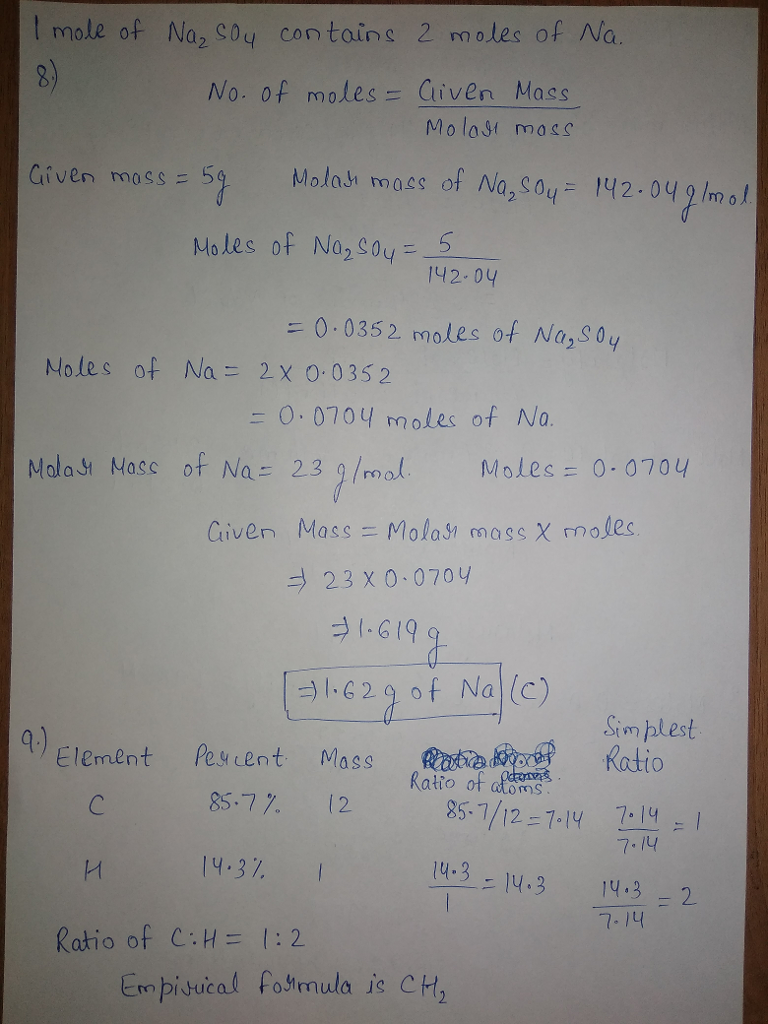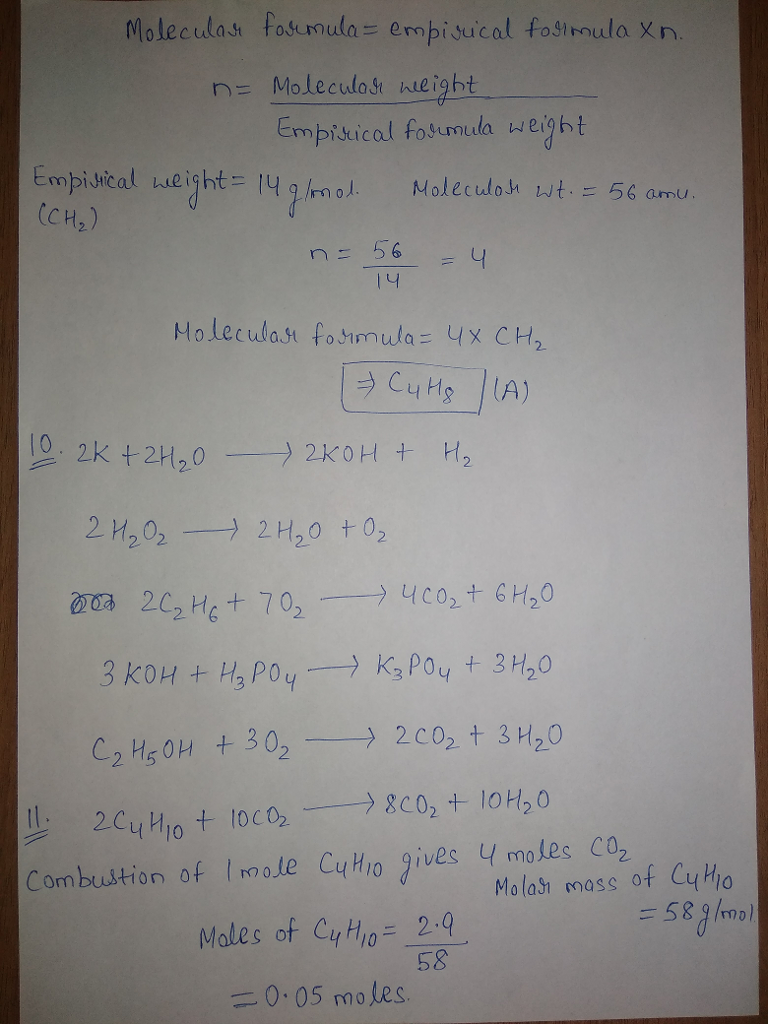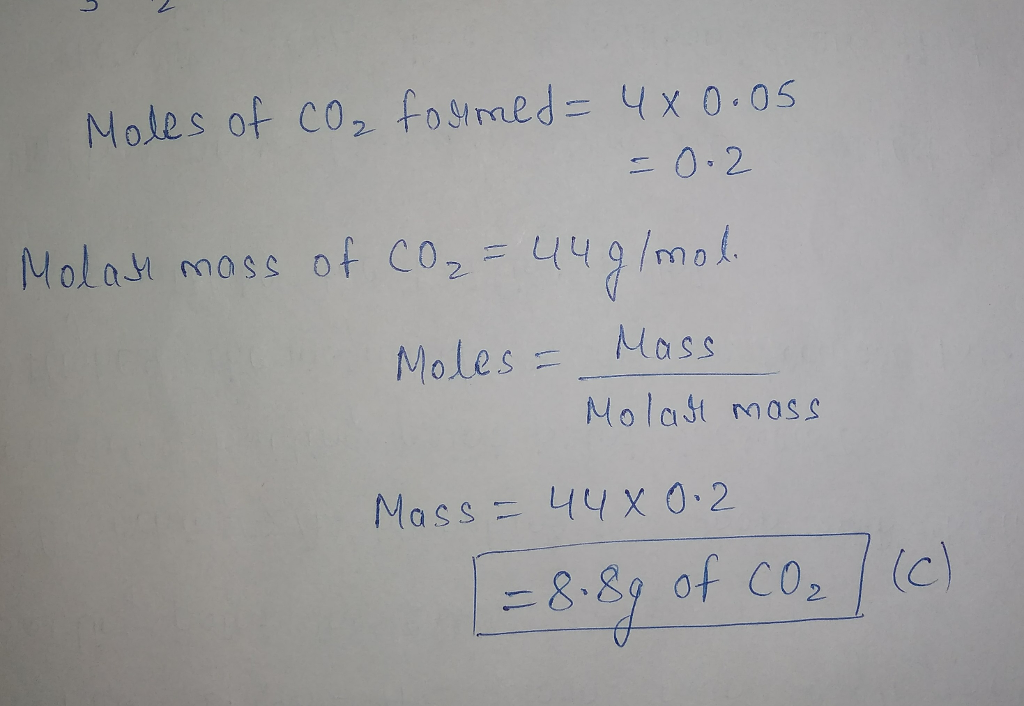#### Earn Coins

Coins can be redeemed for fabulous gifts.

Similar Homework Help Questions
• ### 1. For the reaction 3 H2(g) + N2(g) → 2 NH, (g), 3 mol H2 is...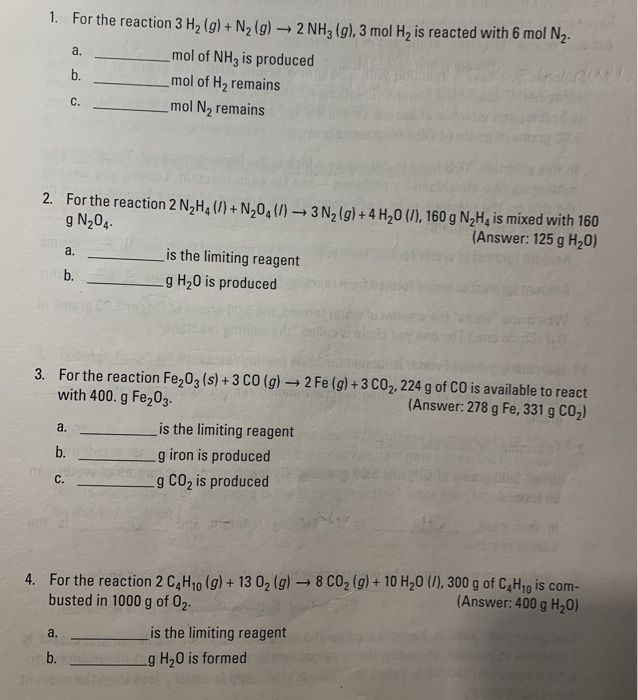1. For the reaction 3 H2(g) + N2(g) → 2 NH, (g), 3 mol H2 is reacted with 6 mol N2- mol of NH3 is produced mol of Hy remains _mol Ny remains 2. For the reaction 2 N H (1) + N20 (1) 3N2(g) + 4 H 0 (), 160 g N,H, is mixed with 160 g N204 (Answer: 125 g H20) is the limiting reagent _g H2O is produced 3. For the reaction Fe203 (s) + 3 CO(g)...

• ### 12. Propane (C3H8) is used as a fuel in many gas grills. The balanced equation for the combustion of C3Hg is shown...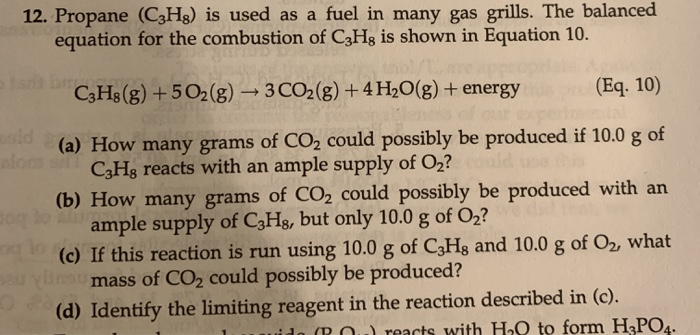12. Propane (C3H8) is used as a fuel in many gas grills. The balanced equation for the combustion of C3Hg is shown in Equation 10. C3H8(g) +502(g) + 3 CO2(g) + 4H2O(g) + energy (Eq. 10) (a) How many grams of CO2 could possibly be produced if 10.0 g of CzHg reacts with an ample supply of Oz? (b) How many grams of CO2 could possibly be produced with an ample supply of C3Hg, but only 10.0 g of O2?...

• ### d. How many moles of O2 must react to produce 0.250 mol of NO? e. How...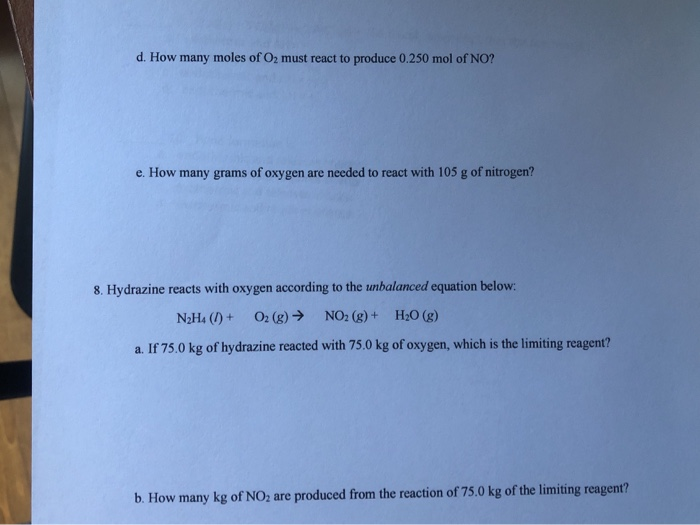d. How many moles of O2 must react to produce 0.250 mol of NO? e. How many grams of oxygen are needed to react with 105 g of nitrogen? 8. Hydrazine reacts with oxygen according to the unbalanced equation below: N2H4 (1) + O2(g) → NO2 (g)+ H2O(g) a. If 75.0 kg of hydrazine reacted with 75.0 kg of oxygen, which is the limiting reagent? b. How many kg of NO2 are produced from the reaction of 75.0 kg of...

• ### Ammonia reacts with oxygen to form nitric oxide and water vapor: 4NH3 + 5O2 ---> 4NO...

Ammonia reacts with oxygen to form nitric oxide and water vapor: 4NH3 + 5O2 ---> 4NO + 6H2O When 20.0 g NH3 and 50.0 g O2 are allowed to react, which is the limiting reagent? A) NO B) O2 C) H2O D) no reagent is limiting E) NH3 What is the coefficient for O2 when the following combustion reaction of a hydrocarbon is balanced? ___C7H14 + ___ O2 ---> ___ CO2 + __ H2O A) none of these B) 10...

• ### A reaction is done by dissolving 5.687 g sodium phosphate and 7.533 g of calcium chloride...

A reaction is done by dissolving 5.687 g sodium phosphate and 7.533 g of calcium chloride in water. These react to form solid calcium phosphate and sodium chloride (this remains dissolved in water). a) Write a balanced equation for the reaction; don’t forget states of matter b) How many grams of calcium phosphate are expected to form if all the sodium phosphate reacts? c) How many grams of calcium phosphate are expected to form if all the calcium chloride reacts?...

• ### 6. Cyclohexane reacts with oxygen to produce carbon dioxide and water by the following balanced chemical...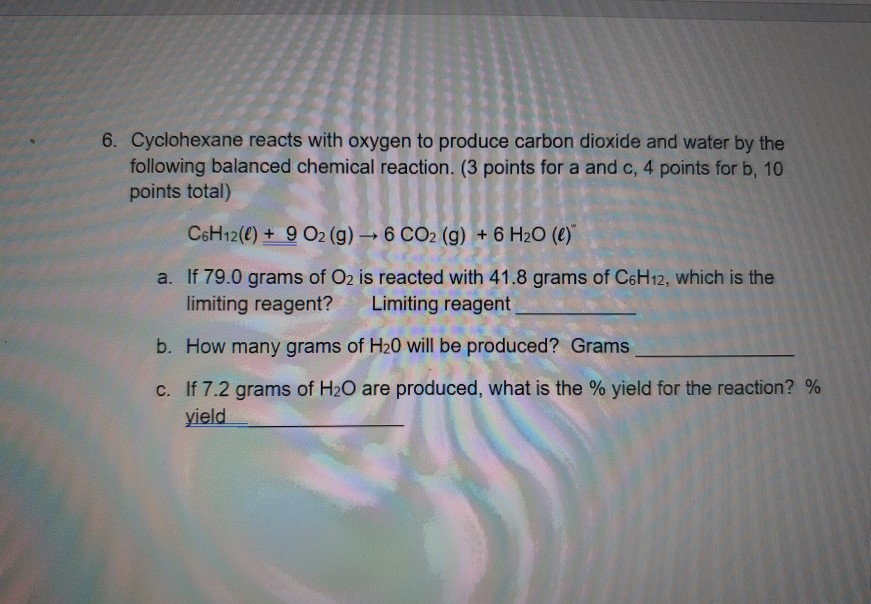6. Cyclohexane reacts with oxygen to produce carbon dioxide and water by the following balanced chemical reaction. (3 points for a and c, 4 points for b. 10 points total) CoH12(C) + 9 O2 (g) - 6 CO2 (g) + 6 H20 (0)" a. If 79.0 grams of O2 is reacted with 41.8 grams of C6H12, which is the limiting reagent? Limiting reagent b. How many grams of H20 will be produced? Grams C. If 7.2 grams of H20 are...

• ### Part A Determine the balanced chemical equation for this reaction. C8H18(g)+O2(g)?CO2(g)+H2O(g) Enter the coefficients for each...

Part A Determine the balanced chemical equation for this reaction. C8H18(g)+O2(g)?CO2(g)+H2O(g) Enter the coefficients for each compound in order, separated by commas. For example, 1,2,3,4 would indicate one mole of C8H18, two moles of O2, three moles of CO2, and four moles of H2O. 2,25,16,18 SubmitHintsMy AnswersGive UpReview Part Correct It is important to balance a chemical equation before using it for calculations. Checking that equations are balanced will help you avoid many errors in chemistry problems. Balanced chemical equation...

• ### Need answerings for A and B and how to (c) 17.0g (d) 32.4 g (e) 14.1g...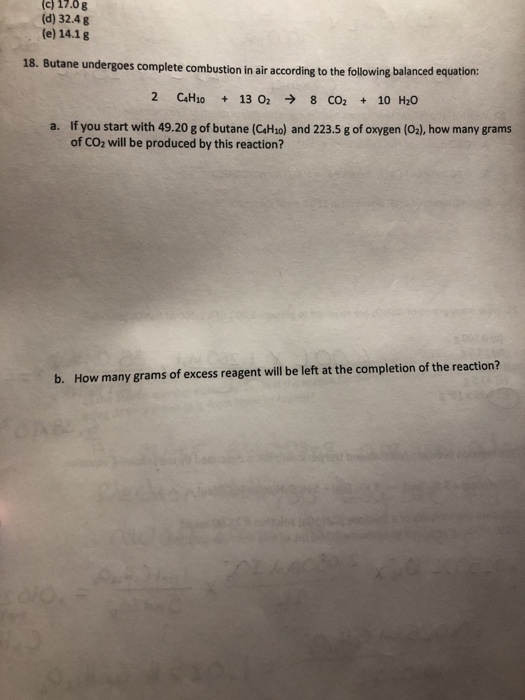Need answerings for A and B and how to (c) 17.0g (d) 32.4 g (e) 14.1g 18. Butane undergoes complete combustion in air according to the following balanced equation: a. If you start with 49.20 g of butane (CaHo) and 223.5 g of oxygen (02), how many grams of CO2 will be produced by this reaction? b. How many grams of excess reagent will be left at the completion of the reaction?

• ### D Question 3 3 pts (This is the same reaction from #2.) You repeat the Diels-Alder...

D Question 3 3 pts (This is the same reaction from #2.) You repeat the Diels-Alder reaction from lab using 3.4 g of maleic anhydride (98.06 g/mol) and 2.5 g of cyclopentadiene (66.1 g/mol) to form the final solid product (164.16 g/mol). All compounds are in a 1:1 ratio in the balanced equation. Assuming all the limiting reagent reacts, how much of the other reagent is there in excess? 0.469 g 0 0.309 g O 1.71 g O 0.208 g...

• ### all please, no mid way answers because then i dont get the help necessary. Name 2)...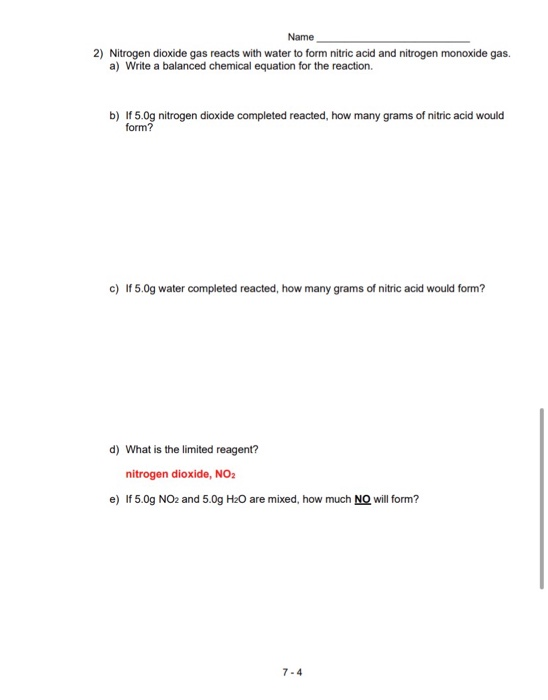all please, no mid way answers because then i dont get the help necessary. Name 2) Nitrogen dioxide gas reacts with water to form nitric acid and nitrogen monoxide gas a) Write a balanced chemical equation for the reaction. b) If 5.Og nitrogen dioxide completed reacted, how many grams of nitric acid would form? c) If 5.0g water completed reacted, how many grams of nitric acid would form? d) What is the limited reagent? nitrogen dioxide, NO2 e) If 5.0g...